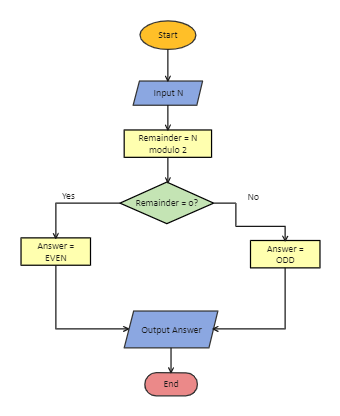Template Community / Output Answer Algorithm FlowchartCharlotte
Published on 2021-07-16
Edit OnlineCreate an algorithm to find whether a number is even or odd. The first step in the algorithm is taking a number as input from the user. The new value of N = 6. The next step in the algorithm (number%2==0), in this step (number%2), returns the remainder after dividing the number by 2. It should be noted here that % is known as modulus, which returns the remainder value after division. The next step, i.e., remainder == 0, simply checks if the remainder value is equal to 0 or not. So if the remainder is 0, then the number is said to be Even else. It is said to be an Odd number.
Tag
algorithm flowchart
Report
0
286PostRecommended Templates